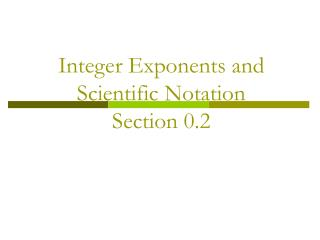DownloadDownload PresentationInteger Exponents and Scientific Notation Section 0.2

# Integer Exponents and Scientific Notation Section 0.2

Download Presentation## Integer Exponents and Scientific Notation Section 0.2

- - - - - - - - - - - - - - - - - - - - - - - - - - - E N D - - - - - - - - - - - - - - - - - - - - - - - - - - -
##### Presentation Transcript

1. Integer Exponents and Scientific NotationSection 0.2

2. What’s an exponent? Exponents are shorthand notation for repeated multiplication: 5555 = 54 There are four 5’s being multiplied together. In 54 , the 5 is called the base and the 4 is the power or exponent. In 5555 , the 5’s are called factors. 

3. Evaluating expressions Evaluating an expression means to find out what it’s worth (giving it’s value)…just do the math. Evaluate the following: (note that the location of the negative sign and the parenthesis make a difference in the answer!) 

4. Evaluating expressions continued Evaluate the following: 3234 This can become: 333333 or: 36 Which is: 729 This idea is called the Product Property of Exponents. When you are multiplying exponentials with the same base you add the exponents. Just remember the bases MUST be the same. 

5. More properties of exponents This can become: Remember that a number divide by itself is 1… So all that is left is 55 which is 25. This is the Quotient Properties of Exponents. When you divide exponentials with the same base, subtract the exponents. 

6. More properties of exponents Power property of exponents: 

7. a. (–4 25)2 = (– 4)2(25)2 –1 115 b. 118 = 1331 = 113 EXAMPLES Evaluate numeric expressions 

8. Simplifying Algebraic Expressions Algebraic expressions are simplified when the following things have happened or are “done”: • All parenthesis or grouping symbols have been eliminated • A base only appears once • No powers are raised to other powers • All exponents are positive 

9. = b–4 +6+7 = b9 r–2–3 ( r –2 )–3 ( s3 )–3 = b. s3 r 6 = s–9 c. 16m4n –5 2n–5 EXAMPLES Simplify algebraic expressions Product of powers property a. b–4b6b7 Power of a quotient property Power of a power property = r6s9 Negative exponent property Quotient of powers property = 8m4n –5 – (–5) = 8m4n0= 8m4 Zero exponent property 

10. (x–3y3)2 x5y6 x –6y6 = x5y6 EXAMPLE Standardized Test Practice SOLUTION The correct answer is B 

11. More Examples

12. More Examples

13. 7z4 y2 GUIDED PRACTICE Simplify the expression. Tell which properties of exponents you used. x–6x5 x3 x2 ;Product of powers property ANSWER (7y2z5)(y–4z–1) ; Product of powersproperty, Negative exponent property ANSWER 

14. x4y–2 3 s 3 2 t–4 x3y6 s6t8 x3 y24 GUIDED PRACTICE ANSWER ; Power of a power property, Negative exponent property ; Quotient of powers property, Power of a Quotient property, Negative exponent property ANSWER 

15. Scientific Notation Scientific Notation was developed in order to easily represent numbers that are either very large or very small. Following are two examples of large and small numbers. They are expressed in decimal form instead of scientific notation to help illustrate the problem 

16.  A very large number: The Andromeda Galaxy (the closest one to our Milky Way galaxy) contains at least 200,000,000,000 stars. A very small number: On the other hand, the weight of an alpha particle, which is emitted in the radioactive decay of Plutonium-239, is0.000,000,000,000,000,000,000,000,006,645 kilograms. As you can see, it could get tedious writing out those numbers repeatedly. So, a system was developed to help represent these numbers in a way that was easy to read and understand: Scientific Notation. 

17. Decimal to Scientific Notation • Move the decimal point so the number shown is between 1 and 10 • Count the number of spaces moved and this is the exponent on the 10 • If the original number is bigger than 1, the exponent is positive • If the original number is between 0 and 1, then the exponent is negative.

18. What to do for scientific notation Write in scientific notation: 200,000,000,000 So we write the number in scientific notation as 2.0 x 1011 Write in scientific notation: 0.000,000,000,000,000,000,000,000,006,645 6.645 x 10-27 

19. Scientific Notation to Decimal • The number of spaces moved is the exponent on the 10 • Move to the right if the exponent is positive • Move to the left if the exponent is negative 6.45 x 104 = 64,500 2.389 x 10-6 = .000002389